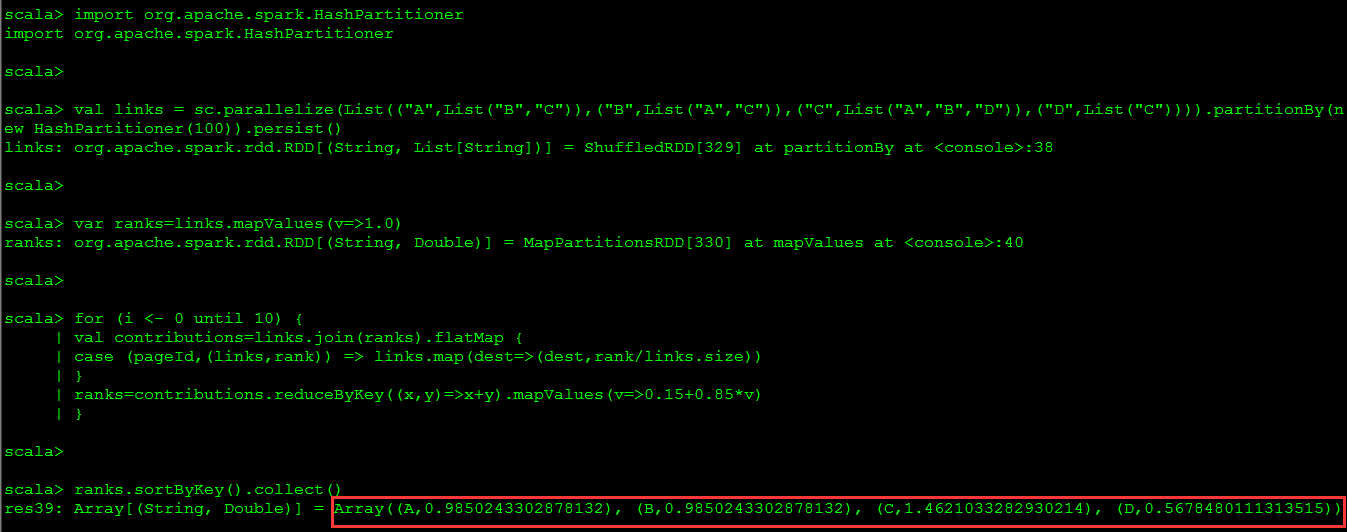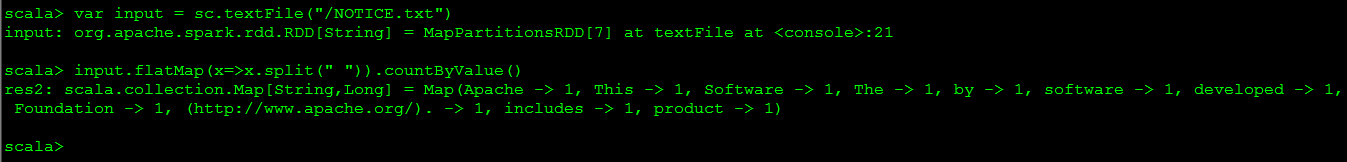# PageRank算法在spark上的简单实现

spark 1.5.0

1. 将每个页面的排序值初始化为1.0。
2. 在每次迭代中，对页面p，向其每个相邻页面（有直接链接的页面）发送一个值为rank(p)/numNeighbors(p)的贡献值。
3. 将每个页面的排序值设为0.15 + 0.85 * contributionsReceived。
最后两个步骤会重复几个循环，在此过程中，算法会逐渐收敛于每个页面的实际PageRank值。在实际操作中，收敛通常需要大约10轮迭代。

A：B C
B：A C
C：A B D
D：C

import org.apache.spark.HashPartitioner

for (i <- 0 until 10) {
}
ranks=contributions.reduceByKey((x,y)=>x+y).mapValues(v=>0.15+0.85*v)
}

ranks.sortByKey().collect()
执行结果如下图所示。Array[(String, List[String])] = Array((A,List(B, C)), (B,List(A, C)), (C,List(A, B, D)), (D,List(C)))
ranksRDD：
Array[(String, Double)] = Array((A,1.0), (B,1.0), (C,1.0), (D,1.0))

contributionsRDD：
Array[(String, Double)] = Array((A,0.5), (A,0.3333333333333333), (B,0.5), (B,0.3333333333333333), (C,0.5), (C,0.5), (C,1.0), (D,0.3333333333333333))
ranksRDD：
Array[(String, Double)] = Array((A,0.8583333333333333), (B,0.8583333333333333), (C,1.8499999999999999), (D,0.43333333333333335))

PR(A)=0.15 + 0.85 * (1/2 + 1/3) = 0.858333
PR(B)=0.15 + 0.85 * (1/2 + 1/3) = 0.858333
PR(C)=0.15 + 0.85 * (1/2 + 1/2 + 1/1) = 1.85
PR(D)=0.15 + 0.85 * (1/3) = 0.433333

PR(A)=0.15 + 0.85 * (0.858333/2 + 1.85/3) = 1.038958191100
PR(B)=0.15 + 0.85 * (0.858333/2 + 1.85/3) = 1.038958191100
PR(C)=0.15 + 0.85 * (0.858333/2 + 0.858333/2 + 0.433333/1) = 1.247916100000
PR(D)=0.15 + 0.85 * (1.85/3) = 0.67416667

PR(A)=0.15 + 0.85 * (1.038958191100/2 + 1.247916100000/3) = 0.945133459550833333
PR(B)=0.15 + 0.85 * (1.038958191100/2 + 1.247916100000/3) = 0.945133459550833333
PR(C)=0.15 + 0.85 * (1.038958191100/2 + 1.038958191100/2 + 0.67416667/1) = 1.606156131935000000
PR(D)=0.15 + 0.85 * (1.247916100000/3) = 0.503576228333333333

虽然代码本身很简单，这个示例程序还是做了不少事情来确保RDD以比较高效的方式进行分区，以最小化通信开销：

scala这语言是真的很简洁，大数据上的通用示例程序wordcount，用scala写一行搞定，如下图所示：

var input = sc.textFile("/NOTICE.txt")
input.flatMap(x=>x.split(" ")).countByValue()07-264万+02-239573
09-21742
05-11546
02-217089
04-102803
11-201397
07-173601